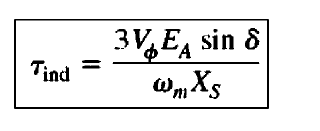Load angle is nothing but an angle different between stator axis and rotor pole axis of the synchronous motor. For ideal motor, the load angle is zero since the rotor poles aligned with stator poles, but in practice, this is not possible. The motor has both mechanical and electrical losses, hence load angle is always present in the synchronous motor.

Load angle can be calculated by using the below-mentioned formula,Refer above, the induced torque is directly proportional to the load angle δ.

It is denoted by δ

It is also called a power angle, torque angle and coupling angle.

Note: Synchronous motor is a constant speed motor which speed does not vary with respect to load.

Look at fig 1.1, The phase voltage V and back emf Eb has some slight difference. This slight difference is called the load angle. At no-load condition, the load angle is very small since the losses in the motor are less.